# 13.13: Correlation and mutual information

•• Contributed by Peter Woolf et al.
• Assistant Professor (Chemical Engineering) at University of Michigan

Authors: Lilian Ngobi, Daniel Wheeler, Daniel McClure, Jeremy Goldfarb

Stewards: Jeff Falta, Taylor Lebeis, Shawn Mayfield, Marc Stewart, Tom Welch

## 13.1 Introduction

The application of control networks to engineering processes requires an understanding of the relationships between system variables. One would expect, for example, to find a relationship between the steam flow rate to a heat exchanger and the outlet stream temperature. How, then, are engineers to quantify the degree of relation between a pair of variables? Often times, investigations into the correlation and mutual information of data sets can provide the answer. Correlation analysis provides a quantitative means of measuring the strength of a linear relationship between two vectors of data. Mutual information is essentially the measure of how much “knowledge” one can gain of a certain variable by knowing the value of another variable. By utilizing these techniques, engineers can increase the selectivity and accuracy of their control systems.

## 13.2 Correlation

### 13.2.1 Population Correlation Coefficient

The correlation coefficient is an important measure of the relationship between two random variables. Once calculated, it describes the validity of a linear fit. For two random variables, X and Y, the correlation coefficient, ρxy, is calculated as follows:

$\rho_{x y}=\frac{\operatorname{cov}(X, Y)}{\sigma_{x} \sigma_{y}}$

That is, the covariance of the two variables divided by the product of their standard deviations. Covariance serves to measure how much the two random variables vary together. This will be a positive number if both variables consistently lie above the expected value and will be negative if one tends to lie above the anticipated value and the other tends to lie below. For a further description of covariance, as well as how to calculate this value, see Covariance on Wikipedia.

The correlation coefficient will take on values from 1 to -1. Values of 1 and -1 indicate perfect increasing and decreasing linear fits, respectively. As the population correlation coefficient approaches zero from either side, the strength of the linear fit diminishes. Approximate ranges representing the relative strength of the correlation are shown below. See the Correlation article on wikipedia for more detailed information about the correlation theory establishing these ranges. The ranges also apply for negative values between 0 and -1.

Correlation

Small: 0.10 to 0.29

Medium: 0.30 to 0.49

Large: 0.50 to 1.00

### 13.2.2 Sample Correlation Coefficient

A modified form of the expression for the correlation coefficient describes the linearity of a data sample. For n measurements of variables X and Y, the sample correlation coefficient is calculated as follows:where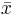andare the sample averages, and sx and sy are the samples’ standard deviations. This correlation coefficient will still take on values from 1 to -1, corresponding to the strength of linearity in the same way as the population correlation coefficient. This expression is often the more useful for process controls applications; an engineer can measure two variables, calculate their means and standard deviations, and ultimately determine the validity of a linear relationship between the two. This method is extremely useful when applied to a sample of experimental data that can be modeled by a normal distribution function. It is simpler and easier to use compared to the population correlation coefficient because it uses only well defined statistical inputs, mean and standard deviation, rather than the complex covariance function.

### 13.2.3 Correlation Coefficient Assumptions: Linearity, Normal Distribution

The correlation coefficient indicates the strength of a linear relationship between two variables with random distribution; this value alone may not be sufficient to evaluate a system where these assumptions are not valid.

The image below shows scatterplots of Anscombe's quartet, a set of four different pairs of variables created by Francis Anscombe. The four y variables have the same mean (7.5), standard deviation (4.12), correlation coefficient (0.81) and regression line (y = 3 + 0.5x).[Image from en.wikipedia.org/wiki/Correlation Correlation]

However, as can be seen on the plots, the distribution of the variables is very different.

The scatterplot of y1 (top left) seems to exhibit random distribution. It corresponds to what one would expect when considering two variables linearly correlated and following normal distribution.

The scatterplot of y2 (top right) does not exhibit random distribution; a plot of its residuals would not show random distribution. However, an obvious non-linear relationship between the two variables can be observed.

The scatterplot of y3 (bottom left) seems to exhibit a near perfect linear relationship with the exception of one outlier. This outlier exerts enough influence to lower the correlation coefficient from 1 to 0.81. A Q test should be performed to determine if a data point should be retained or rejected.

The scatterplot of y4 (bottom right) shows another example when one outlier is enough to produce a high correlation coefficient, even though the relationship between the two variables is not linear.

These examples indicate that the correlation coefficient alone should not be used to indicate the validity of a linear fit.

### 13.2.4 Engineering Applications

Measuring the correlation of system variables is important when selecting manipulated and measured control pairs. Say, for instance, that an engineer takes several measurements of the temperature and pressure within a reactor and notes that the data pairs have a correlation coefficient of approximately one. The engineer now understands that by applying a control system to maintain the temperature, he will also be controlling the reactor pressure by way of the linear relationship between the two variables.

Here we begin to see the role correlations play in defining MIMO versus SISO systems. Generally, engineers seek control systems with independent pairs of manipulated (u) and measured variables (x). If two inputs strongly influence a single output as measured by the correlation value, then the system will be difficult to decouple into a SISO controller. Correlation values closer to 0 for all but one manipulated variable means the system will be easier to decouple because here a single system input controls a single system output and there is limited effect on other process variables.

### 13.2.5 Correlation in Mathematica

Correlation coefficients can be quickly calculated with the aid of Mathematica. To illustrate by example, suppose that an engineer measures a series of temperatures inside a bacterial chemostat and the corresponding protein concentration in the product. She measures temperatures of 298, 309, 320, 333, and 345 K corresponding to protein concentrations of 0.4, 0.6, 0.7, 0.85, and 0.9 weight percent protein (these values are for illustrative purposes and do not reflect the performance of an actual chemostat). The engineer enters these measurements into Mathematica as a pair of vectors as follows: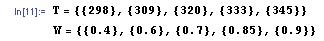where T is a vector containing the temperature measurements and C is a vector containing the concentration measurements. To determine the correlation coefficient, simply use the syntax below.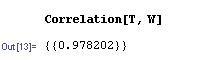In this case, the correlation coefficient of nearly 1 indicates a strong degree of linearity between the chemostat temperature and the protein concentration, informing the engineer that she can essentially control this concentration through temperature control. The sample correlation function in Mathematica uses an approximate solution with the mean and standard deviation of the data set, but the value is very close to the true correlation function value. This can be seen in the figure below as the dashed line represents the sample correlation function, which deviates slightly from the true correlation function. As the sample becomes larger (i.e. higher n) the approximation gets closer to the true value. This represents a limitation of the sample correlation function and demonstrates how Mathematica can be used to determine multiple correlations between sets of data.## 13.3 Mutual Information

Mutual information (also referred to as transinformation) is a quantitative measurement of how much one random variable (Y) tells us about another random variable (X). In this case, information is thought of as a reduction in the uncertainty of a variable. Thus, the more mutual information between X and Y, the less uncertainty there is in X knowing Y or Y knowing X. For our purposes, within any given process, several parameters must be selected in order to properly run the process. The relationship between variables is integral to correctly determine working values for the system. For example, adjusting the temperature in a reactor often causes the pressure to change as well. Mutual information is most commonly measured in logarithms of base 2 (bits) but is also found in base e (nats) and base 10 (bans).

### 13.3.1 Explanation of Mutual Information

The mathematical representation for mutual information of the random variables A and B are as follows:where,

p(a,b) = joint probability distribution function of A and B.

p(a) = marginal probability distribution function of A.

p(b) = marginal probability distribution function of B.

For a review and discussion of probability distribution functions, please refer to Probability Distribution (Wikipedia).

When referring to continuous situations, a double integral replaces the double summation utilized above.where,

p(a,b) = joint probability density function of A and B.

p(a) = marginal probability density function of A.

p(b) = marginal probability density function of B.

For a review and discussion of probability density functions, please refer to Probability Density Function (Wikipedia).

By performing the functions above, one measures the distance between the joint distribution/density functions of A and B. Since mutual information is always measuring a distance, the value obtained from this calculation will always be nonnegative and symmetric. Therefore,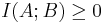In both cases, the information shared between both A and B is being measured. If A and B are independent of each other, then the difference between the two would be zero. In terms of chemical engineering processes, two independent variables would share no mutual information which means that one variable provides no information about the other variable. If A and B were identical, then all the information derived from obtaining variable A would supply the knowledge needed to get variable B.

By knowing the mutual information between two variables A and B, modeling of a multivariable system can be accomplished. If two or more variables provide the same information or have similar effects on one outcome, then this can be taken into consideration while constructing a model. For example, if the level of a tank was observed to be too high, pressure and temperature could be plausible variables. If manipulating both of these variables causes a change in the fluid level in the tank, then there exists some mutual information between the pressure and temperature. It would then be important to establish the relationship between temperature and pressure to appropriately control the level of fluid in the tank.

### 13.3.2 Visual Representation of Mutual InformationFigure 1. This figure demonstrates how y provides information for x. The blue is p(x) and the red is p(x|y) for a specific y.

A. y shares mutual information with x such that given a y1 the values of x are now narrowed down to the five values.

B. y shares mutual information with x but it shares less information than A and therefore is not as helpful as A, as it does not eliminate as many potential x values.

C. y does not share any mutual information. Given the y3 value, no x values are eliminated as potential values. Since y3 does not provide any information there is no information between the two variables.

D. y shares mutual information with x. Because of the bimodal distribution, one more piece of information is required to describe which distribution lies within. Therefore, D gives as much information as part B. B gives 11 potential values while D gives 10 potential values. However, one additional bit of information is required to describe which of the two curves the x values lie on, thus there are 11 needed inputs.

### 13.3.3 Relating Mutual Information to Other Quantities/Concepts

Mutual information can be related to other concepts to gain a comprehensive understanding of the information obtained from any given parameter. Mutual information discussions are most commonly coupled with a discussion on Shannon entropy (See Shannon Entropy for more information on entropy).

Relative Entropy

In terms of probability theory, the relative entropy of a system measures the distance between two distinct probability functions. In chemical engineering applications, mutual information can be used to determine the entropy inherent in a system. Mutual information in this aspect can now be defined simply as:where,

H(A) = marginal entropy of A.

H(B) = marginal entropy of B.

H(A | B) = conditional entropy of A.

H(B | A) = conditional entropy of B.

H(A,B) = joint entropy of A and B.

As described above, the marginal entropy still represents the uncertainty around a given variable A and B. Conditional entropy describes the uncertainty in the specified variable that remains after the other variable is known. In terms of mutual information, the conditional entropies of A and B tell us that a certain number of bits needs to be transferred from A in order to determine B and vice versa. Once again, these bits of information are determined by the logarithmic base chosen for analysis. One can determine the uncertainty in several different process parameters with respect to any other process variable.

Mutual Information and Boolean Models

Recall that via BooleanModels, a complex series of sensors and valves can be modeled into a simple control system. A Boolean variable, however, will never return a value because it can only return True or False. This knowledge can be combined to determine a relationship between two different variables (from Boolean model) and then their dependency can be quantified (mutual information). Utilizing the same logic that goes into constructing truth tables, data can be input into tables to determine the mutual information value.

## 13.4 Correlation Example

Suppose an engineer wants to test the applicability of the ideal gas law to a closed tank containing a fixed amount of his company’s gaseous product. Given that the vessel is of constant volume, and given that no change in moles will occur in this fixed system, one would hypothesize that the ideal gas law would predict a linear relationship between the temperature and pressure within the tank.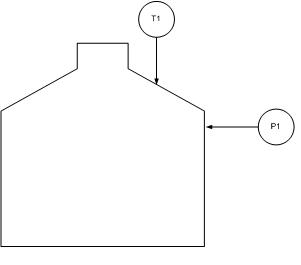The engineer makes the following measurements and notes his findings in Excel.Before proceeding with his analysis, the engineer decides to calculate the correlation coefficient for these values in Mathematica.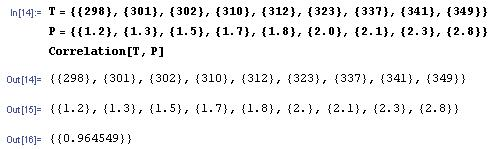Noting the correlation coefficient’s approximate value of 1, the engineer proceeds by creating a linear fit of the data in Excel.Indeed, the data are nearly linear.

## 13.5 Summary

If an engineer were trying to determine if two random variables are linearly related, calculating correlation coefficients would be the first step to determine this. If the mean and standard deviation for both variables are known values (i.e. calculated from thousands of measurements), then the population correlation coefficient should be used. To determine the correlation coefficient for a smaller set of data, the sample correlation coefficient equations should be used. If the two variables were shown to have a high degree of correlation, the next step would be to plot the data and graphically check the linear relationship of this data. A high correlation coefficient does not necessarily mean the two variables are linearly related as shown by Anscombe’s quartet, so this graphical check is necessary.

Mutual information helps reduce the range of the probability density function (reduction in the uncertainty) for a random variable X if the variable Y is known. The value of I(X;Y) is relative, and the larger its value, the more information that is known of X. It is generally beneficial to try to maximize the value of I(X;Y), thus minimizing uncertainty. The concept of mutual information is quite complex and is the basis of information theory.